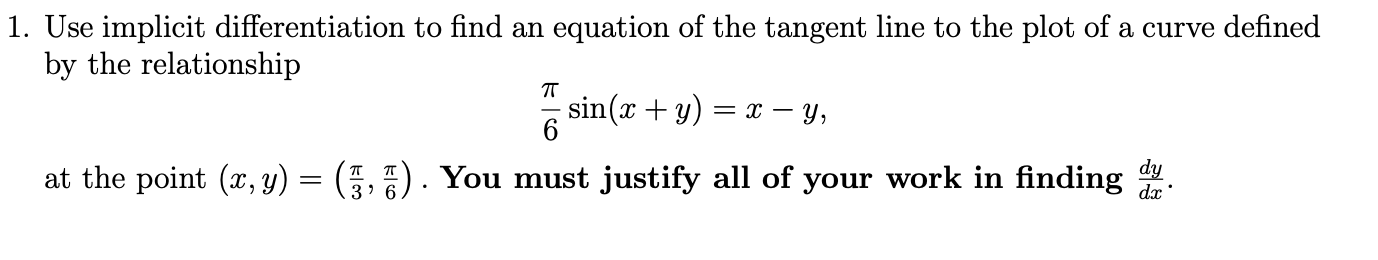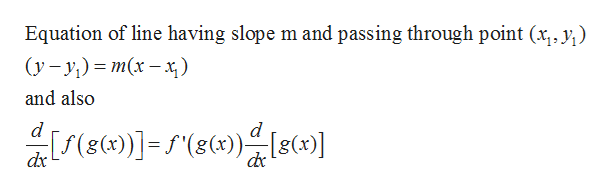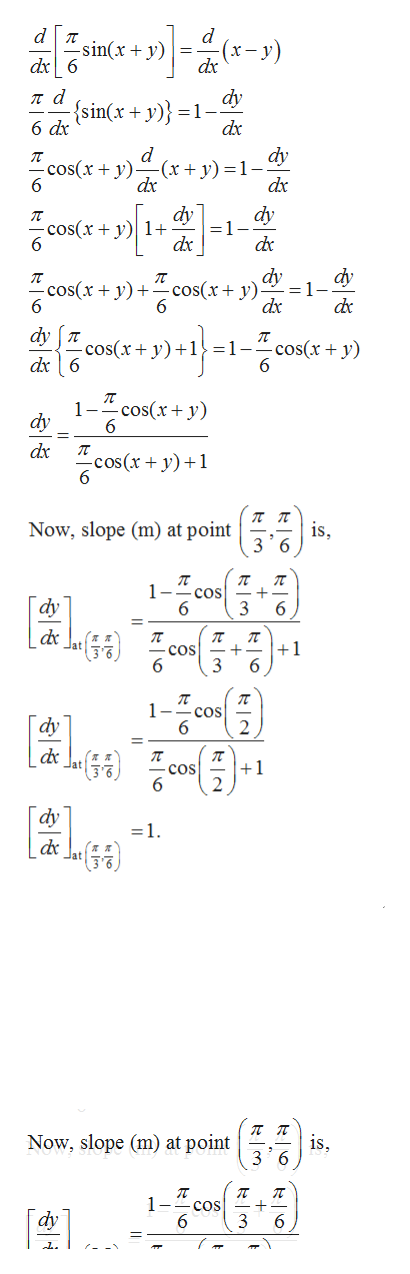# 1. Use implicit differentiation to find an equation of the tangent line to the plot of a curve definedby the relationshipT6dydat the point (x, y) = (3, 7). You must justify all of your work in finding

Question
6 views

Please justify stuff that is asked. thank you have a wonderful day :)help_outlineImage Transcriptionclose1. Use implicit differentiation to find an equation of the tangent line to the plot of a curve defined by the relationship T 6 dy d at the point (x, y) = (3, 7). You must justify all of your work in finding fullscreen
check_circle

Step 1

Given function and point is

Step 2

Using formulahelp_outlineImage TranscriptioncloseEquation of line having slope m and passing through point (x, y) (у - у) %3D т(х — х) and also d d dx fullscreen
Step 3

First find the slope of the tangent line to the curve at given poi...help_outlineImage Transcriptionclose-sin(x + y) dx6 dy ла {sin(x + y)} =1- 6 dx dx dy d -(x+ y) = 1 - dx -cos(x + y 6 dx dy =1-- dy cos(x y1 dx dy dy =1 dx cos(x y)sx+ y): 6 dc 6 dy T dx6 cos(x y os(x+ y)+1} =1- 1-Tcos(+y) dy 6 dx cos(x+y) is 3 6 Now, slope (m) at point 1- cos 6 dy 6 п cos 3 п +1 6 6 1 - cos dy +1 dy 1 л п is Now, slope (m) at point 1- cos 6 dy 6 + fullscreen

### Want to see the full answer?

See Solution

#### Want to see this answer and more?

Solutions are written by subject experts who are available 24/7. Questions are typically answered within 1 hour.*

See Solution
*Response times may vary by subject and question.
Tagged in

### Other